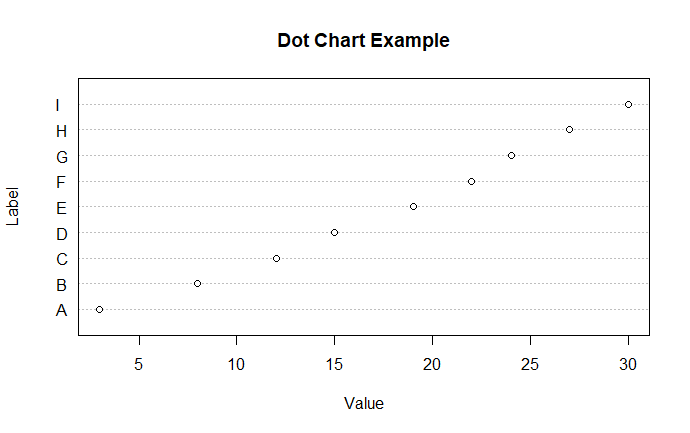Open In App

# Create Dot Charts in R Programming – dotchart () Function

dotchart() function in R Language is used to create a dot chart of the specified data. A dot chart is defined as a plot which is used to draw a Cleveland dot plot.

Syntax: dotchart(x, labels = NULL, groups = NULL, gcolor = par(“fg”), color = par(“fg”)) Parameters: x: it is defined as numeric vector or matrix labels: a vector of labels for each point. groups: a grouping variable indicating how the elements of x are grouped. gcolor: color to be used for group labels and values. color: the color(s) to be used for points and labels.

Example 1:

## r

 `# Dot chart of a single numeric vector``dotchart``(mtcars\$mpg, labels = ``row.names``(mtcars),``         ``cex = 0.9, xlab = "mpg")`

## r

 `# Plot and color by groups cyl``grps <- ``as.factor``(mtcars\$cyl)``my_cols <- ``c``("blue", "darkgreen", "orange")``dotchart``(mtcars\$mpg, labels = ``row.names``(mtcars),``         ``groups = grps, gcolor = my_cols,``         ``color = my_cols[grps],``         ``cex = 0.9,  pch = 22, xlab = "mpg")`

Example :

## R

 `# Create a vector of data``data <- ``c``(3, 8, 12, 15, 19, 22, 24, 27, 30)` `# Create a vector of labels for the data points``labels <- ``c``(``"A"``, ``"B"``, ``"C"``, ``"D"``, ``"E"``, ``"F"``, ``"G"``, ``"H"``, ``"I"``)` `# Create a dot chart``dotchart``(data, labels = labels, main = ``"Dot Chart Example"``, xlab = ``"Value"``, ylab = ``"Label"``)`

output :